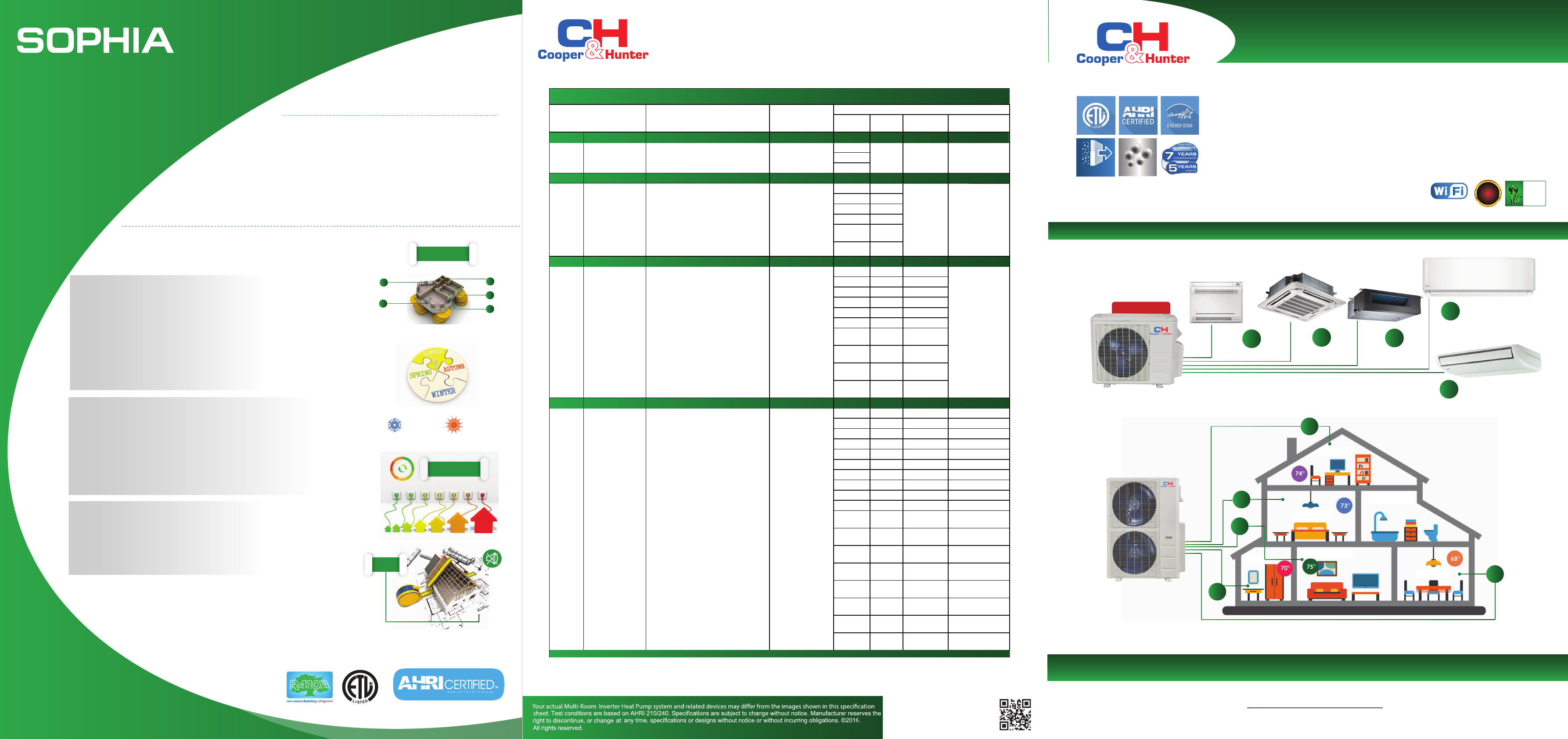# Sophia BookletMatching Combinations and Capacities
C&H
Inverter ductless systems.
or visit us at
cooperandhunter
.
us
cold
catalyst filter
SILVER ION
SOPHIA MULTI ZONE
Multi-Room Inverter Heat Pump Solution
Bedrooms | Living Rooms | Dining Rooms | Home Ofﬁces | Sunrooms | Basements | Computer / Server Rooms
www.cooperandhunter.us
GREEN
FIN
GOLD
FIN
UP TO 5 ZONES
3
4
2
1
Console Type
Duct Type
Floor and Ceiling
Ceiling Cassette
2
3
Wall Mount
4
5
Up to 22.5 SEER
Attic
Hall
Living Room
Kitchen
Bathroom
Bedroom
5
1
*
* selected models only
Multi-Room Inverter Heat
Pump Mini-Splits: Complete
Climate Solution For
Year-Round Cooling
and Heating
Mini splits have two main components — an outdoor
compressor/condenser and an indoor air-handling unit. A
conduit, which houses the power cable, refrigerant pipes, and a
condensate drain, links the outdoor and indoor units. The indoor
units are available in dierent shapes and capacities for easy
mix-and-match.
What is a Ductless Mini-Split?
Benefits:
1
2
3
5
4
up to 5 zones
СOLD
WARM
High Energy Eciency
- Mini splits have no ducts, so they avoid the energy losses associated with the
ductwork of central forced air systems
- Duct losses can account for up to 30% of energy consumption
up to 30%
45-60
feet
Year-Round Solution
- Heat pumps provide heat when it is cold and air conditioners provide
cooling when it is warm
Easy Installation and Comfort
- Small size and exibility for zoning
- A multi-zone system splits a house or oce into multiple zones that can be
controlled separately
- Heat pump units can heat & cool up to 5 zones
- The owner can choose between several indoor unit styles for easy installation
and tting room design
Quiet Operation
- The ductless heat pump has low noise level. The outdoor unit can be located
as far as 45-60 feet from the indoor unit.
- This makes it possible to cool rooms on the front side of a house but locate
the compressor in a more advantageous or inconspicuous place on the outside
of the building.
Two units
Three
units
Four units Five units
9+9
9+12
12+12
9+9 9+9+9
9+12 9+9+12
9+18 9+9+18
12+12 9+12+12
12+18 12+12+12
18+18
9+9 9+9+9 9+9+9+9
9+12 9+9+12 9+9+9+12
9+18 9+9+18 9+9+9+18
9+24 9+12+12 9+9+12+12
12+12 9+12+18 9+9+12+18
12+18 9+18+18 9+12+12+12
12+24 12+12+12 12+12+12+12
18+18 12+12+18
12+18+18
12+12+24
9+9 9+9+9 9+9+9+9 9+9+9+9+9
9+12 9+9+12 9+9+9+12 9+9+9+9+12
9+18 9+9+18 9+9+9+18 9+9+9+9+18
9+24 9+9+24 9+9+9+24 9+9+9+9+24
12+12 9+12+12 9+9+12+12 9+9+9+12+12
12+18 9+12+18 9+9+12+18 9+9+9+12+18
12+24 9+12+24 9+9+12+24 9+9+9+18+18
18+18 9+18+18 9+9+18+18 9+9+12+12+12
18+24 9+18+24 9+9+18+24 9+9+12+12+18
24+24 9+24+24 9+12+12+12 9+12+12+12+12
12+12+12 9+12+12+18 9+12+12+12+18
12+12+18 9+12+12+24 12+12+12+12+12
12+12+24 9+12+18+18
12+18+18 9+18+18+18
12+18+24 12+12+12+12
12+24+24 12+12+12+18
18+18+18 12+12+12+24
18+18+24 12+12+18+18
Wall m
ounted: 9K/12K/18K;
Cassette/Duct/Console&Floor
Ceiling: 9K/12K/
18K;
1 drive 4
1 drive 5
Wall
mounted:
9K/12K/18K/24K;
Cassette/Duct/Console&Floor
Ceiling: 9K/12K/18K/24K;
Wall mounted:
9+9+9+9+9
Duct:
9+9+9+9+9
1drive 2
CH18MSPH230VO
Wall mounted: 9K/12K;
Cassette/Duct/Console: 9K/12K;
1 drive 3
Wall mounted: 9K/12K/18K;
Cassette/Duct/Console&Floor
Ceiling: 9K/12K/18K;
Multi DC Outdoor Unit Avaliable Indoor
Standard
Combination
Wall mounted:
9+9+9+9
Duct:
9+9+9+9
Wall mounted:
9+9+9
Duct:
9+9+9
Wall mounted:
9+9
Duct:
9+9
CH28MSPH230VO
CH36MSPH230VO
CH48MSPH230VO
There should be only one Floor Ceiling or Duct unit.
If total indoor units load exceeds nominal capacity of outdoor unit, the practical output capacity of every indoor unit will be correspondingly
attenuated. This situation is very evidently during heating mode.
None:
Rules: Outdoor unit Capacity*0.66<= Indoor unit total capacity <= Outdoor unit Capacity 1.33